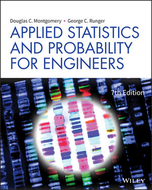×
Get Full Access to Applied Statistics And Probability For Engineers - 7 Edition - Chapter 12.4 - Problem 12.4.3
Get Full Access to Applied Statistics And Probability For Engineers - 7 Edition - Chapter 12.4 - Problem 12.4.3

×ISBN: 9781119409533 2045

## Solution for problem 12.4.3 Chapter 12.4

Applied Statistics and Probability for Engineers | 7th Edition

• Textbook Solutions
• 2901 Step-by-step solutions solved by professors and subject experts
• Get 24/7 help from StudySoup virtual teaching assistantsApplied Statistics and Probability for Engineers | 7th Edition

4 5 1 344 Reviews
14
0
Problem 12.4.3

Consider the soil absorption data in Exercise 12.1.4.

a. Find 95% confidence intervals on the regression coefficients.

b. Find a 95% confidence interval on the mean soil absorption index when $$x_{1}$$ = 200 and $$x_{2}$$ = 50.

c. Find a 95% prediction interval on the soil absorption index when $$x_{1}$$ = 200 and $$x_{2}$$ = 50.

Text Transcription:

x_1

x_2

Step-by-Step Solution:
Step 1 of 3

STA 215 Chapter 8 Regression Wisdom Looking at residuals is the easiest way to check for linearity. Residuals reveal subtleties not apparent from the scatterplot. They can also help us focus on the appropriateness of the regression line. Example: Below is a scatter plot of the Dive Heart Rate (beats per minute) and the Duration (minutes) of dives by emperor penguins. This also includes a residual plot. Are the assumptions for linear regression (discussed in Chapter 7) met Discuss. th Intro Stats, 4 Edition, by Richard D. De Veaux, Paul F. Velleman, and David E. Bock, 2014. Example: Nutritionists, concerned about “empty calories” in breakfast cereal, recorded facts about 77 cereals, including their calories per serving and sugar content (in grams). The scatterplot and residual plot are below. Are the assumptions for linear regression met Discuss. Below is a histogram of the residuals for the breakfast cereal data. What features of this histogram may cause concern that the scatterplot and residual plot did not alert us to Intro Stats, 4 Edition, by Richard D. De Veaux, Paul F. Velleman, and David E. Bock, 2014. As it turns out, the high residual cereals are what you would call the “healthy cereals.” Most of these contain both fruit and nuts and

Step 2 of 3

Step 3 of 3

##### ISBN: 9781119409533

This textbook survival guide was created for the textbook: Applied Statistics and Probability for Engineers, edition: 7. The full step-by-step solution to problem: 12.4.3 from chapter: 12.4 was answered by Aimee Notetaker, our top Statistics solution expert on 05/05/22, 11:14AM. Applied Statistics and Probability for Engineers was written by Aimee Notetaker and is associated to the ISBN: 9781119409533. The answer to “?Consider the soil absorption data in Exercise 12.1.4. a. Find 95% confidence intervals on the regression coefficients. b. Find a 95% confidence interval on the mean soil absorption index when $$x_{1}$$ = 200 and $$x_{2}$$ = 50.c. Find a 95% prediction interval on the soil absorption index when $$x_{1}$$ = 200 and $$x_{2}$$ = 50.Text Transcription:x_1x_2” is broken down into a number of easy to follow steps, and 56 words. Since the solution to 12.4.3 from 12.4 chapter was answered, more than 203 students have viewed the full step-by-step answer. This full solution covers the following key subjects: . This expansive textbook survival guide covers 112 chapters, and 30 solutions.

Unlock Textbook Solution